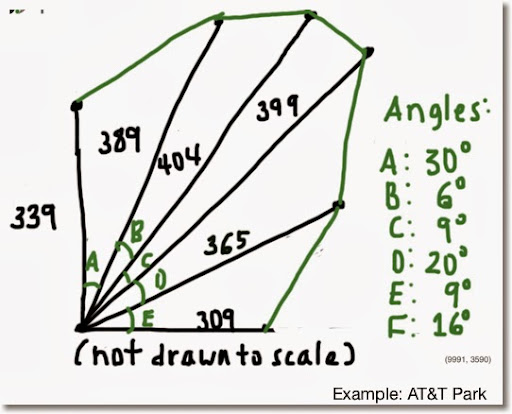## Saturday, January 11, 2014

### HP Prime: Area of a Fan-Shaped Field (Baseball Field)Given the lines of various length from a single vertex and the angles between the lines, the area of the field can be calculated.

FANAREA(list of lengths, list of angles in degrees)

The result is a list of two elements.
First element: area of the field
Second element: list: length of the connecting lines

Example:
AT&T Park (see the above figure)
Dimensions from Clem's Blog:
http://www.andrewclem.com/Baseball/ATTPark.html

Angles measured with a protractor. So, realistically, my estimate of area is rough.

L1:={339,382,404,399,421,365,309}
L2:={30,6,9,20,9,16}
FANAREA(L1,L2) returns
{109337.870804, {191.1175010192, 46.6352856001, 63.1995292625,
144.030366611, 83.1849883392, 108.96879942}}

Area of the field: approximately 109,337.871

Note: CHAR(10) gives a line break in a string.

PROGRAM:

// Declare Subs
SUB1();
SUB2();

EXPORT FANAREA(L1,L2);
BEGIN
// 2014-01-11 EWS
// Area of a field
// lines from vertex w/angles
LOCAL I,T:=0,L3:=L2;
// Degree
HAngle:=1;

// Validate
IF SIZE(L1)≠SIZE(L2)+1 THEN
MSGBOX("Error: Wrong"+CHAR(10)
+"Size"+CHAR(10)
+"L2≠L1+1");
KILL; END;

// Calculation
FOR I FROM 1 TO SIZE(L2) DO
L3(I):=SUB1(L1(I),L1(I+1),L2(I));
T:=T+SUB2(L1(I),L1(I+1),L3(I));
END;

MSGBOX("Area: "+T);
RETURN {T, L3};
END;

// Law of Cosines
SUB1(A,B,C)
BEGIN
RETURN √(A^2+B^2-2*A*B*COS(C));
END;

//Heron's Formula
SUB2(A,B,C)
BEGIN
LOCAL S:=(A+B+C)/2;
RETURN √(S*(S-A)*(S-B)*(S-C));
END;

#### 1 comment:

1.